# Operator ergodic theorem

(diff) ← Older revision | Latest revision (diff) | Newer revision → (diff)

A general name for theorems on the limit of means along an unboundedly lengthening "time interval", or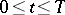, for the powers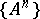of a linear operatoracting on a Banach space (or even on a topological vector space, see ), or for a one-parameter semi-group of linear operatorsacting on(cf. also Ergodic theorem). In the latter case one can also examine the limit of means along an unboundedly diminishing time interval (local ergodic theorems, see , ; one also speaks of "ergodicity at zero" , see ). Means can be understood in various senses in the same way as in the theory of summation of series. The most frequently used means are the Cesàro means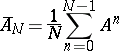or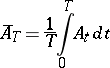and the Abel means, ,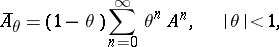or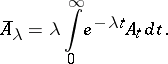The conditions of ergodic theorems automatically ensure the convergence of these infinite series or integrals; under these conditions, although the Abel means are formed by using all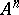or, the values oforin a finite period of time, unboundedly increasing when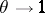(or), play a major part. The limit of the means (, etc.) can be understood in various senses: In the strong or weak operator topology (statistical ergodic theorems, i.e. the von Neumann ergodic theorem — historically the first operator ergodic theorem — and its generalizations), in the uniform operator topology (uniform ergodic theorems, see , , ), while ifis a function space on a measure space, then also in the sense of almost-everywhere convergence of the means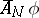, etc., where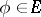(individual ergodic theorems, i.e. the Birkhoff ergodic theorem and its generalizations; see, for example, the Ornstein–Chacon ergodic theorem; these are not always called operator ergodic theorems, however). Some operator ergodic theorems compare the force of various of the above-mentioned variants with each other, establishing that, from the existence of limits of means in one sense, it follows that limits exist in another sense . Some theorems speak not of the limit of means, but of the limit of the ratios of two means (e.g. the Ornstein–Chacon theorem).

There are also operator ergodic theorems for-parameter and even more general semi-groups.

How to Cite This Entry:
Operator ergodic theorem. Encyclopedia of Mathematics. URL: http://encyclopediaofmath.org/index.php?title=Operator_ergodic_theorem&oldid=11719
This article was adapted from an original article by D.V. Anosov (originator), which appeared in Encyclopedia of Mathematics - ISBN 1402006098. See original article